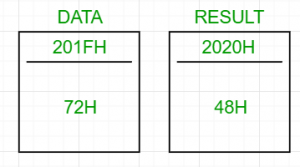GeeksforGeeks App
Open AppBrowser
Continue

# 8085 program to convert a BCD number to binary

Problem – Write an assembly language program for converting a 2 digit BCD number to its binary equivalent using 8085 microprocessor.

Examples:

```Input : 72H (0111 0010)2
Output : 48H (in hexadecimal) (0011 0000)2
((4x16)+(8x1))=72
```Algorithm:

1. Load the BCD number in the accumulator
2. Unpack the 2 digit BCD number into two separate digits. Let the left digit be BCD1 and the right one BCD2
3. Multiply BCD1 by 10 and add BCD2 to it

If the 2 digit BCD number is 72, then its binary equivalent will be
7 x OAH + 2 = 46H + 2 = 48H

Steps:

1. Load the BCD number from the memory location (201FH, arbitrary choice) into the accumulator
2. Temporarily store the accumulator’s value in B
3. Obtain BCD2 by ANDing the accumulator with 0FH and store it in C
4. Restore the original value of the accumulator by moving the value in B to A. AND the accumulator with F0H
5. If the value in the accumulator equals 0, then BCD2 is the final answer and store it in the memory location, 2020H (arbitrary)
6. Else, shift the accumulator to right 4 times to obtain BCD1. Next step is to multiply BCD1 by 0AH
7. Multiplication: Move BCD1 to D and initialise E with 0AH as the counter. Clear the accumulator to 0 and add D to it E number of times
8. Finally, add C to the accumulator and store the result in 2020H

2020H contains the result.

2000HLDA 201FH
2001H
2002H
2003HMOV B, A
2004HANI 0FH
2005H
2006HMOV C, A
2007HMOV A, B
2008HANI F0H
2009H
200AHJZ SKIPMULTIPLY
200BH
200CH
200DHRRC
200EHRRC
200FHRRC
2010HRRC
2011HMOV D, A
2012HXRA A
2013HMVI E, 0AH
2014H